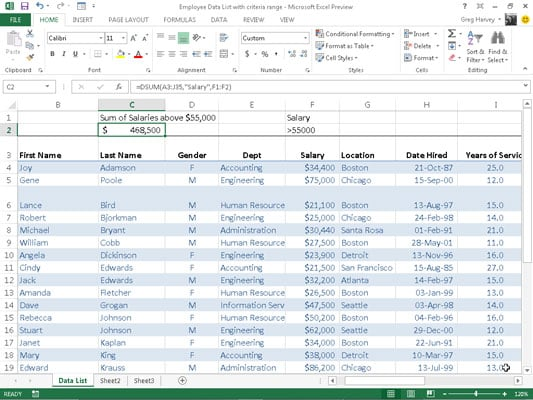##### Excel 2013 All-in-One For DummiesExcel 2013 includes a number of database functions that you can use to calculate statistics, such as the total, average, maximum, minimum, and count in a particular field of the data list only when the criteria that you specify are met.

For example, you could use the DSUM function in an Employee data list to compute the sum of all the salaries for employees who were hired after January 1, 2000, or you could use the DCOUNT function to compute the number of records in the data list for the Human Resources department.

The database functions, regardless of the difference in names (and they all begin with the letter D) and the computations that they perform, all take the same three arguments as illustrated by the DAVERAGE function:

`DAVERAGE(<i>database,field,criteria</i>)`

The arguments for the database functions require the following information:

• database is the argument that specifies the range containing the list and it must include the row of field names in the top row.

• field is the argument that specifies the field whose values are to be calculated by the database function (averaged in the case of the DAVERAGE function). You can specify this argument by enclosing the name of the field in double quotes (as in "Salary" or "Date Hired"), or you can do this by entering the number of the column in the data list (counting from left to right with the first field counted as 1).

• criteria is the argument that specifies the address of the range that contains the criteria that you’re using to determine which values are calculated. This range must include at least one field name that indicates the field whose values are to be evaluated and one cell with the values or expression to be used in the evaluation.

Note that in specifying the field argument, you must refer to a column in the data list that contains numeric or date data for all the database functions with the exception of DGET. All the rest of the database functions can’t perform computations on text fields.

If you mistakenly specify a column with text entries as the field argument for these database functions, Excel returns an error value or 0 as the result. The table lists the various database functions available in Excel along with an explanation of what each one calculates. (You already know what arguments each one takes.)

The Database Functions in Excel
Database Function What It Calculates
DAVERAGE Averages all the values in a field of the data list that match the criteria you specify.
DCOUNT Counts the number of cells with numeric entries in a field of the data list that match the criteria you specify.
DCOUNTA Counts the number of nonblank cells in a field of the data list that match the criteria you specify.
DGET Extracts a single value from a record in the data list that matches the criteria you specify. If no record matches, the function returns the #VALUE! error value. If multiple records match, the function returns the #NUM! error value.
DMAX Returns the highest value in a field of the data list that matches the criteria you specify.
DMIN Returns the lowest value in a field of the data list that matches the criteria you specify.
DPRODUCT Multiplies all the values in a field of the data list that match the criteria you specify.
DSTDEV Estimates the standard deviation based on the sample of values in a field of the data list that match the criteria you specify.
DSTDEVP Calculates the standard deviation based on the population of values in a field of the data list that match the criteria you specify.
DSUM Sums all the values in a field of the data list that match the criteria you specify.
DVAR Estimates the variance based on the sample of values in a field of the data list that match the criteria you specify.
DVARP Calculates the variance based on the population of values in a field of the data list that match the criteria you specify.

The Database functions are too rarely used to rate their own command button on the Ribbon’s Formulas tab. As a result, to use them in a worksheet, you must click the Function Wizard (fx) button on the Formula bar and then select Database from the Select a Category drop-down list box and then click the function to use or type the Database function directly into the cell.

Cell C2 in the worksheet shown in this figure contains the following formula:`=DSUM(A3:J35,"Salary",F1:F2)`

This DSUM function computes the total of all the salaries in the data list that are above \$55,000. This total is \$468,500, as shown in cell C2, which contains the formula.

To perform this calculation, specify the range A3:J35, which contains the entire data list. This range includes the top row of field names as the database argument (which Excel 2013 automatically converted to its range name equivalent, Table2[#All]).

Then specify "Salary" as the field argument of the DSUM function because this is the name of the field that contains the values that you want totaled. Finally, specify the range E1:E2 as the criteria argument of the DSUM function because these two cells contain the criteria range that designate that only the values exceeding 55000 in the Salary field are to be summed.